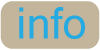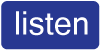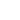# How to say maths calculations in English exercise

Being able to say calculations in English is very useful. Maths is used not only in business, but also in everyday life. It is very common that people learn the vocabulary of calculations when they are starting to learn English, but then forgot it because most calculations are written on paper and not spoken.

In this online exercise we will look at the English vocabulary of maths calculations, focusing on what the symbols used are called and how to explain and say basic calculations.

## Exercise: How to say calculations

Read the following conversation between Peter and Juan, where Peter helps Juan with English vocabulary for calculations and maths.

Focus on the names of these different mathematical symbols and calculations which are in bold and then do the quiz at the end to check that you both understand their meaning and remember them.

Juan:'I know I should know this, but how do you say this symbol ' - ' in a calculation in English?'

Peter:'In English we call the ' - ' symbol minus, for example, 6 minus 3. You can also say the same calculation in a different way by using a verb and saying subtract 6 by 3.'

Juan:'And the ' + ' symbol?'

Peter:'The ' + ' symbol is called plus, for example, 7 plus 2 is 9. You can also say the same calculation in a different way by using the verb add, for example, add 7 to 2. Another type of calculation is division, which is represented by the symbol ' ÷ '. But when you use it in the middle of a calculation, you say divided by. For example, 6 divided by 3 equals 2. Another way to say this calculation is by saying divide 6 by 3.'

Juan:'I know that the ' × ' symbol is called times in a calculation, like 2 times 4 equals 8. But what is the verb?'

Peter:'We normally use multiply as the verb, for example, multiply 2 by 4. You use these verbs when you are instructing somebody to do a calculation or explaining to them how you did it.'

Juan:'There are a few other things that I'd like to check with you. How do you say a number below zero, for example, -1.052?'

Peter:'You would say minus 1 point zero five two. You need to remember that in English when you say the number that comes after the decimal point '.', you say each number individually. For example, you would say the number 14.315, fourteen point three one five.'

Juan:'Thanks. The last question I have is, say I eat 20 apples in June, 22 apples in July and 26 apples in August. Is there a better of saying, I normally eat 23 apples each month?'

Peter:'Yes, there is. Instead of saying normally, you could say average. So, on average I eat 23 apples per month.'

## Quiz: How to say maths calculations in English

Below is an online quiz with definitions of each of the words/phrases in bold from the above text. Now fill in the blanks with one of these words/phrases in bold. Only use one word/phrase once and write it as it is in the text. Click on the "Check Answers" button at the bottom of the quiz to check your answers.

When the answer is correct, two icons will appear next to the question. The first is an Additional Information Icon "". Click on this for extra information on the word/phrase and for a translation. The second is a Pronunciation Icon "". Click on this to listen to the pronunciation of the word/phrase.

1. A word that has a meaning similar to 'medium', isAverage:(noun) In this context 'average' means the result from adding together several different amounts and then dividing the total by the number of amounts that have been added. It is used with numbers and statistics. It is better to use 'average' than 'medium' when talking about numbers and statistics in English. In Spanish: "medio". Close
 Average: Close

2. When you use the ' × ' symbol in a calculation, you sayTimes:(prep) This preposition is always used between the two numbers in a calculation, e.g. 'six times three'. You can also use 'multiplied by' instead of 'times'. In Spanish: "multiplicado por". Close
 Times: Close

3. The verb for ' - ' in calculations, isSubtract:(verb) The infinitive is 'to subtract'. This is always used in front of both numbers in the calculation. In addition, you always separate the two numbers with 'by', e.g. 'subtract 7 by 3'. This structure is commonly used when you are telling/instructing somebody to do a calculation or explaining how you did a calculation. In Spanish: "restar". Close
 Subtract: Close

4. When you use the ' ÷ ' symbol in a calculation, you sayDivided by:(prep) 'divided by' can be used in two ways in calculations. The first, is when saying or reading out a calculation. In this situation 'divided by' goes between the two numbers in the calculation, e.g. 'six divided by three'. In this situation 'divided by' is a fixed phrase and never changes. The second, is when you are telling/instructing somebody to do a calculation or explaining how you did a calculation. In this situation the verb 'to divide' is always used in front of both numbers in the calculation. In addition, you always separate the two numbers with 'by', e.g. 'divide 9 by 3'. In this use, the verb can change, e.g. 'divided, divide, have divided etc...' In Spanish: "dividido entre". Close
 Divided by: Close

5. The name of the ' - ' symbol when a number is below zero, isMinus:(adjective) With negative values or numbers, the ' - ' symbol is in front of the number. In English, we say minus for the symbol, i.e. '-10' is 'minus 10'. In Spanish: "negativo/menos". Close
 Minus: Close

6. The verb for ' + ' in calculations, isAdd:(verb) The infinitive is 'to add'. This is always used in front of both numbers in the calculation. In addition, you always separate the two numbers with 'to', e.g. 'add 7 to 3'. This structure is commonly used when you are telling/instructing somebody to do a calculation or explaining how you did a calculation. In Spanish: "sumar". Close

7. When you use the ' - ' symbol in a calculation, you sayMinus:(prep) This preposition is always used between the two numbers in a calculation, e.g. 'six minus three'. In Spanish: "menos". Close
 Minus: Close

8. The way to say ' . ' in numbers like 1.5, isPoint:(noun) This is a shortened version of 'decimal point'. When saying a number like 1.5, you always say 'one point five'. The 'point' separates the whole number from the decimal. 'Point' is not used when talking about money. In Spanish: "coma". Close
 Point: Close

9. When you use the ' = ' symbol in a calculation, you sayEquals:(verb) 'equals' is used to give the result of a calculation. It always follows the numbers in a calculation, e.g. 'six – three equals three'. It is generally used as a fixed phrase. Sometimes people use 'is' instead of 'equals', e.g. 'six – three is three'. In Spanish: "son". Close
 Equals: Close

10. The verb for ' × ' in calculations, isMultiply:(verb) The infinitive is 'to multiply'. This is always used in front of both numbers in the calculation. In addition, you always separate the two numbers with 'by', e.g. 'multiply 7 by 3'. This structure is commonly used when you are telling/instructing somebody to do a calculation or explaining how you did a calculation. In Spanish: "multiplicar". Close
 Multiply: Close

11. When you use the ' + ' symbol in a calculation, you sayPlus:(prep) This preposition is always used between the two numbers in a calculation, e.g. 'six plus three'. In Spanish: "más". Close
 Plus: Close

## Practice

Now that you understand the new vocabulary of calculations, practise it by creating your own sentences in English with the new words/phrases.